# 三、卡通化图像

• 如何访问网络摄像头
• 如何在实时视频流中进行键盘和鼠标输入
• 如何创建一个交互式应用
• 如何使用高级图像过滤器
• 如何将图像卡通化

# 访问网络摄像头

import cv2

cap = cv2.VideoCapture(0)

# Check if the webcam is opened correctly
if not cap.isOpened():
raise IOError("Cannot open webcam")

while True:
frame = cv2.resize(frame, None, fx=0.5, fy=0.5, interpolation=cv2.INTER_AREA)
cv2.imshow('Input', frame)

c = cv2.waitKey(1)
if c == 27:
break

cap.release()
cv2.destroyAllWindows()


# 底层原理

while循环的第一行中，我们有以下行：

ret, frame = cap.read()


if c == 27:


# 扩展捕获选项

cap = cv2.VideoCapture(1 + cv2.CAP_QT) // Webcam index 1 + reader implementation QuickTime


# 键盘输入

import cv2

def print_howto():
print("""
Change color space of the
input video stream using keyboard controls. The control keys are:
1\. Grayscale - press 'g'
2\. YUV - press 'y'
3\. HSV - press 'h'
""")

if __name__=='__main__':
print_howto()
cap = cv2.VideoCapture(0)

# Check if the webcam is opened correctly
if not cap.isOpened():
raise IOError("Cannot open webcam")

cur_mode = None
while True:
# Read the current frame from webcam

# Resize the captured image
frame = cv2.resize(frame, None, fx=0.5, fy=0.5, interpolation=cv2.INTER_AREA)

c = cv2.waitKey(1)
if c == 27:
break
# Update cur_mode only in case it is different and key was pressed
# In case a key was not pressed during the iteration result is -1 or 255, depending
# on library versions
if c != -1 and c != 255 and c != cur_mode:
cur_mode = c

if cur_mode == ord('g'):
output = cv2.cvtColor(frame, cv2.COLOR_BGR2GRAY)
elif cur_mode == ord('y'):
output = cv2.cvtColor(frame, cv2.COLOR_BGR2YUV)
elif cur_mode == ord('h'):
output = cv2.cvtColor(frame, cv2.COLOR_BGR2HSV)
else:
output = frame
cv2.imshow('Webcam', output)

cap.release()
cv2.destroyAllWindows()


# 鼠标输入

import cv2
import numpy as np

def detect_quadrant(event, x, y, flags, param):
if event == cv2.EVENT_LBUTTONDOWN:
if x > width/2:
if y > height/2:
point_top_left = (int(width/2), int(height/2))
point_bottom_right = (width-1, height-1)
else:
point_top_left = (int(width/2), 0)
point_bottom_right = (width-1, int(height/2))

else:
if y > height/2:
point_top_left = (0, int(height/2))
point_bottom_right = (int(width/2), height-1)
else:
point_top_left = (0, 0)
point_bottom_right = (int(width/2), int(height/2))

img = param["img"]
# Repaint all in white again
cv2.rectangle(img, (0,0), (width-1,height-1), (255,255,255), -1)
cv2.rectangle(img, point_top_left, point_bottom_right, (0,100,0), -1)

if __name__=='__main__':
width, height = 640, 480
img = 255 * np.ones((height, width, 3), dtype=np.uint8)
cv2.namedWindow('Input window')

while True:
cv2.imshow('Input window', img)
c = cv2.waitKey(1)
if c == 27:
break

cv2.destroyAllWindows()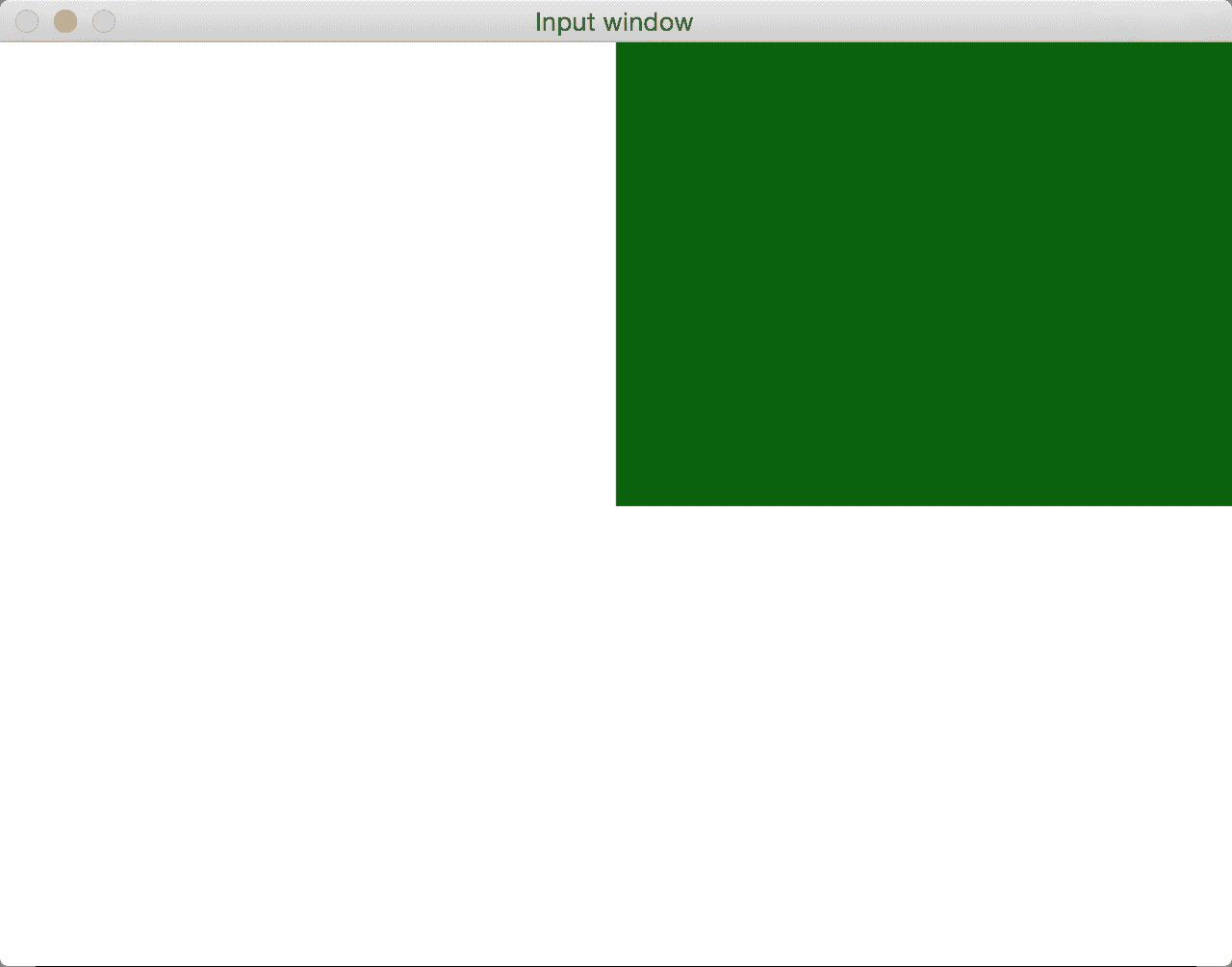# 到底发生了什么？

>>> import cv2
>>> print([x for x in dir(cv2) if x.startswith('EVENT')])


detect_quadrant函数中的第二个和第三个参数提供了鼠标单击事件的XY坐标。 一旦知道了这些坐标，就可以很容易地确定其所在的象限。使用此信息，我们就可以继续使用cv2.rectangle()绘制具有指定颜色的矩形。 这是一个非常方便的函数，它使用左上角和右下角在具有指定颜色的图像上绘制矩形。

# 与实时视频流交互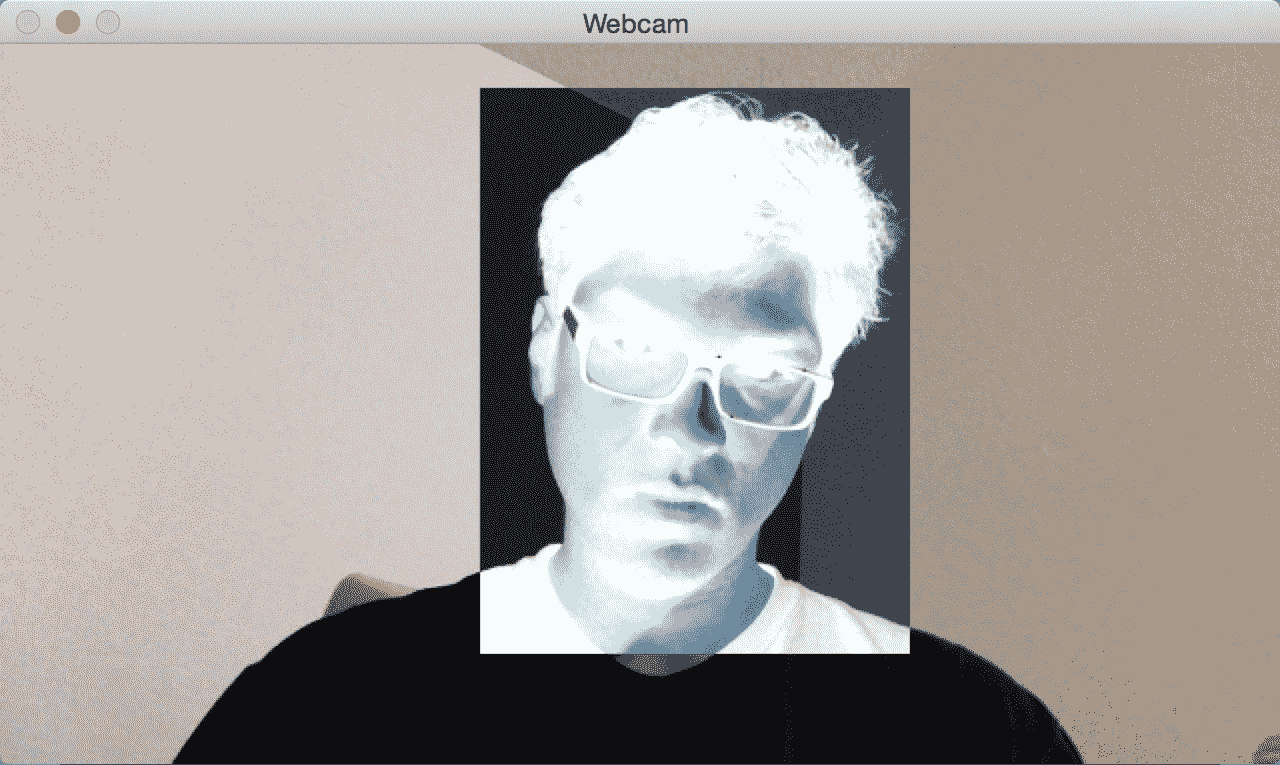import cv2
import numpy as np

def update_pts(params, x, y):
global x_init, y_init
params["top_left_pt"] = (min(x_init, x), min(y_init, y))
params["bottom_right_pt"] = (max(x_init, x), max(y_init, y))
img[y_init:y, x_init:x] = 255 - img[y_init:y, x_init:x]

def draw_rectangle(event, x, y, flags, params):
global x_init, y_init, drawing
# First click initialize the init rectangle point
if event == cv2.EVENT_LBUTTONDOWN:
drawing = True
x_init, y_init = x, y
# Meanwhile mouse button is pressed, update diagonal rectangle point
elif event == cv2.EVENT_MOUSEMOVE and drawing:
update_pts(params, x, y)
# Once mouse botton is release
elif event == cv2.EVENT_LBUTTONUP:
drawing = False
update_pts(params, x, y)

if __name__=='__main__':
drawing = False
event_params = {"top_left_pt": (-1, -1), "bottom_right_pt": (-1, -1)}

cap = cv2.VideoCapture(0)

# Check if the webcam is opened correctly
if not cap.isOpened():
raise IOError("Cannot open webcam")

cv2.namedWindow('Webcam')
# Bind draw_rectangle function to every mouse event
cv2.setMouseCallback('Webcam', draw_rectangle, event_params)

while True:
img = cv2.resize(frame, None, fx=0.5, fy=0.5, interpolation=cv2.INTER_AREA)
(x0,y0), (x1,y1) = event_params["top_left_pt"], event_params["bottom_right_pt"]
img[y0:y1, x0:x1] = 255 - img[y0:y1, x0:x1]
cv2.imshow('Webcam', img)

c = cv2.waitKey(1)
if c == 27:
break

cap.release()
cv2.destroyAllWindows()


# 我们是怎么做的？

cv2.setMouseCallback('Webcam', draw_rectangle, event_params)


# 卡通化图像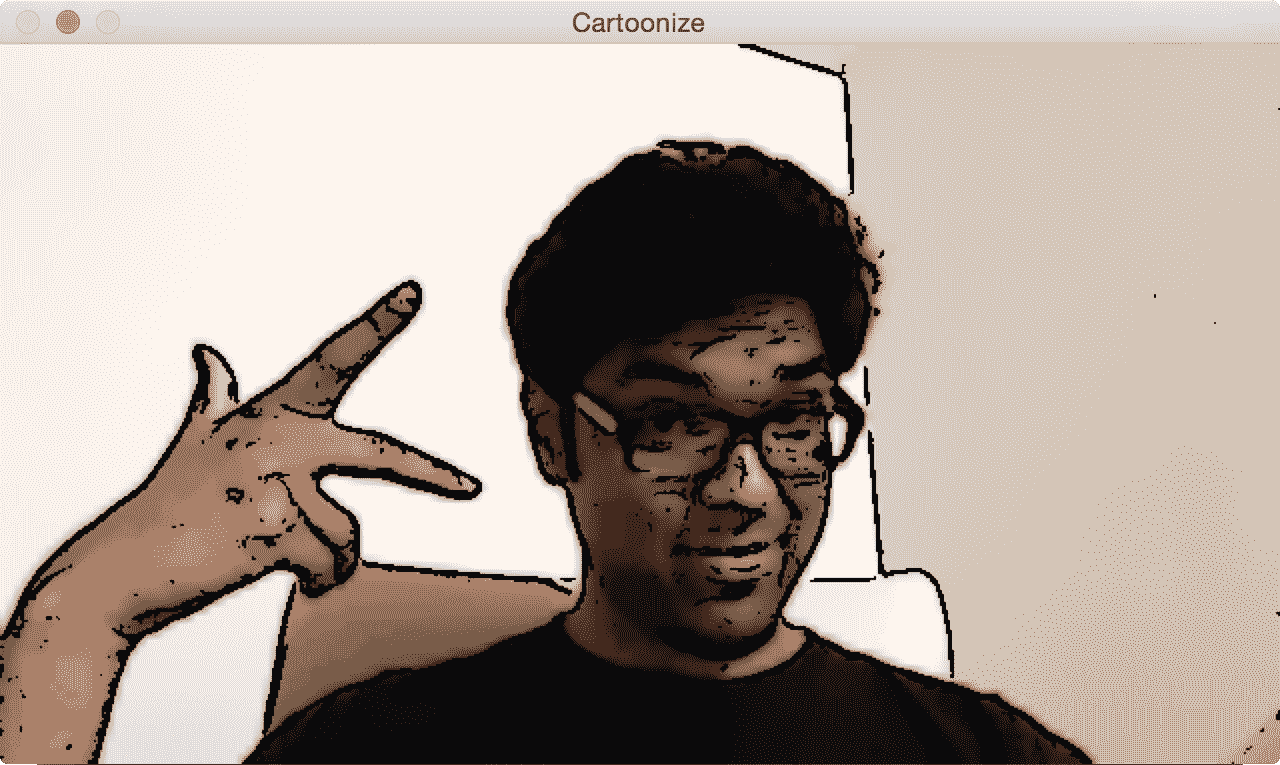import cv2
import numpy as np

def print_howto():
print("""
Change cartoonizing mode of image:
1\. Cartoonize without Color - press 's'
2\. Cartoonize with Color - press 'c'
""")

def cartoonize_image(img, ksize=5, sketch_mode=False):
num_repetitions, sigma_color, sigma_space, ds_factor = 10, 5, 7, 4
# Convert image to grayscale
img_gray = cv2.cvtColor(img, cv2.COLOR_BGR2GRAY)

# Apply median filter to the grayscale image
img_gray = cv2.medianBlur(img_gray, 7)

# Detect edges in the image and threshold it
edges = cv2.Laplacian(img_gray, cv2.CV_8U, ksize=ksize)
ret, mask = cv2.threshold(edges, 100, 255, cv2.THRESH_BINARY_INV)

# 'mask' is the sketch of the image
if sketch_mode:

# Resize the image to a smaller size for faster computation
img_small = cv2.resize(img, None, fx=1.0/ds_factor, fy=1.0/ds_factor, interpolation=cv2.INTER_AREA)

# Apply bilateral filter the image multiple times
for i in range(num_repetitions):
img_small = cv2.bilateralFilter(img_small, ksize, sigma_color, sigma_space)

img_output = cv2.resize(img_small, None, fx=ds_factor, fy=ds_factor, interpolation=cv2.INTER_LINEAR)

dst = np.zeros(img_gray.shape)

# Add the thick boundary lines to the image using 'AND' operator
return dst

if __name__=='__main__':
print_howto()
cap = cv2.VideoCapture(0)

cur_mode = None
while True:
frame = cv2.resize(frame, None, fx=0.5, fy=0.5, interpolation=cv2.INTER_AREA)

c = cv2.waitKey(1)
if c == 27:
break

if c != -1 and c != 255 and c != cur_mode:
cur_mode = c

if cur_mode == ord('s'):
cv2.imshow('Cartoonize', cartoonize_image(frame, ksize=5, sketch_mode=True))
elif cur_mode == ord('c'):
cv2.imshow('Cartoonize', cartoonize_image(frame, ksize=5, sketch_mode=False))
else:
cv2.imshow('Cartoonize', frame)

cap.release()
cv2.destroyAllWindows()


# 解构代码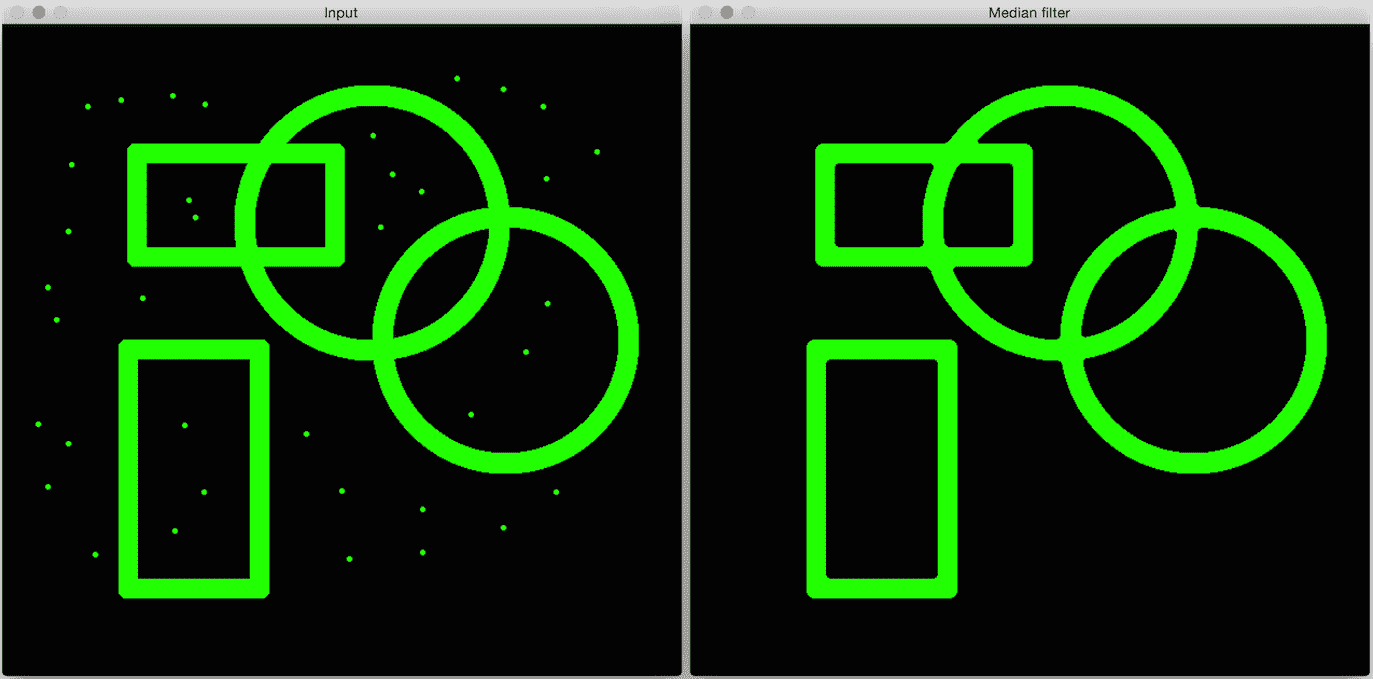import cv2
import numpy as np

output = cv2.medianBlur(img, ksize=7)
cv2.imshow('Input', img)
cv2.imshow('Median filter', output)
cv2.waitKey()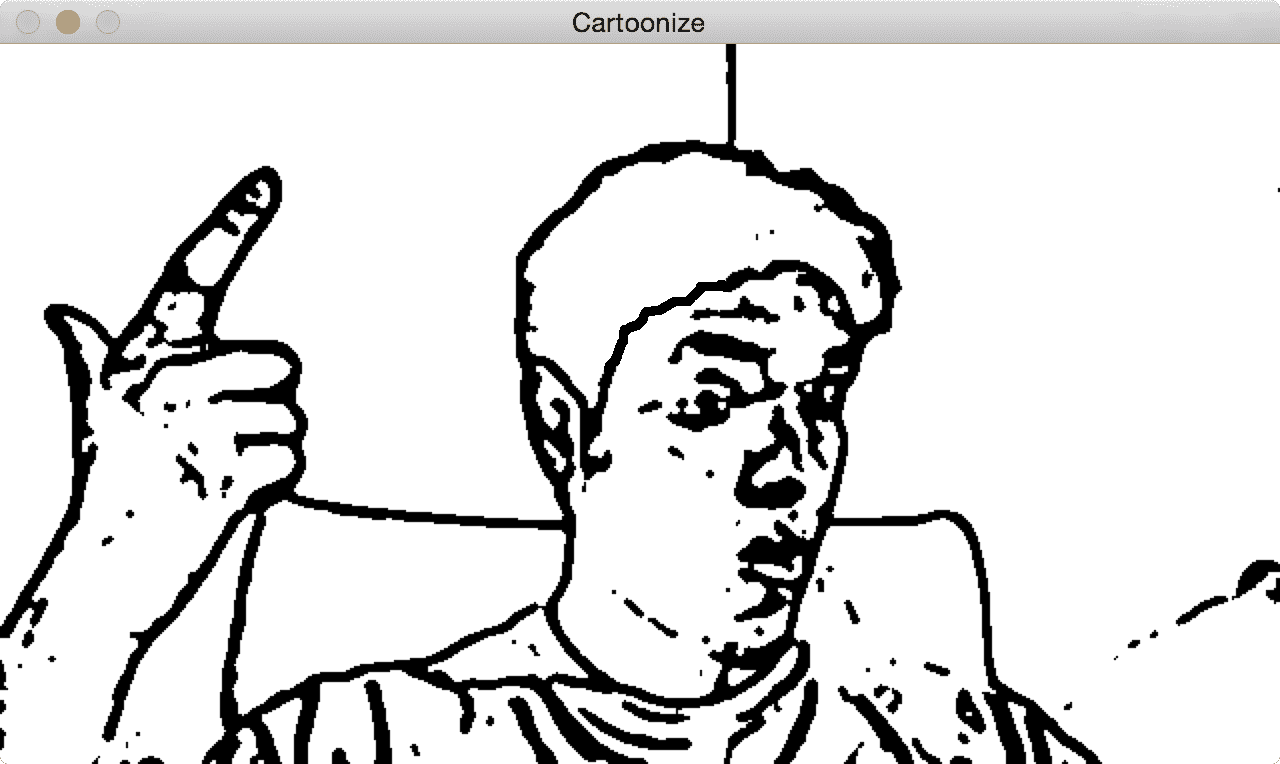if sketch_mode:
kernel = np.ones((3,3), np.uint8)
img_eroded = cv2.erode(img_sketch, kernel, iterations=1)
return cv2.medianBlur(img_eroded, ksize=5)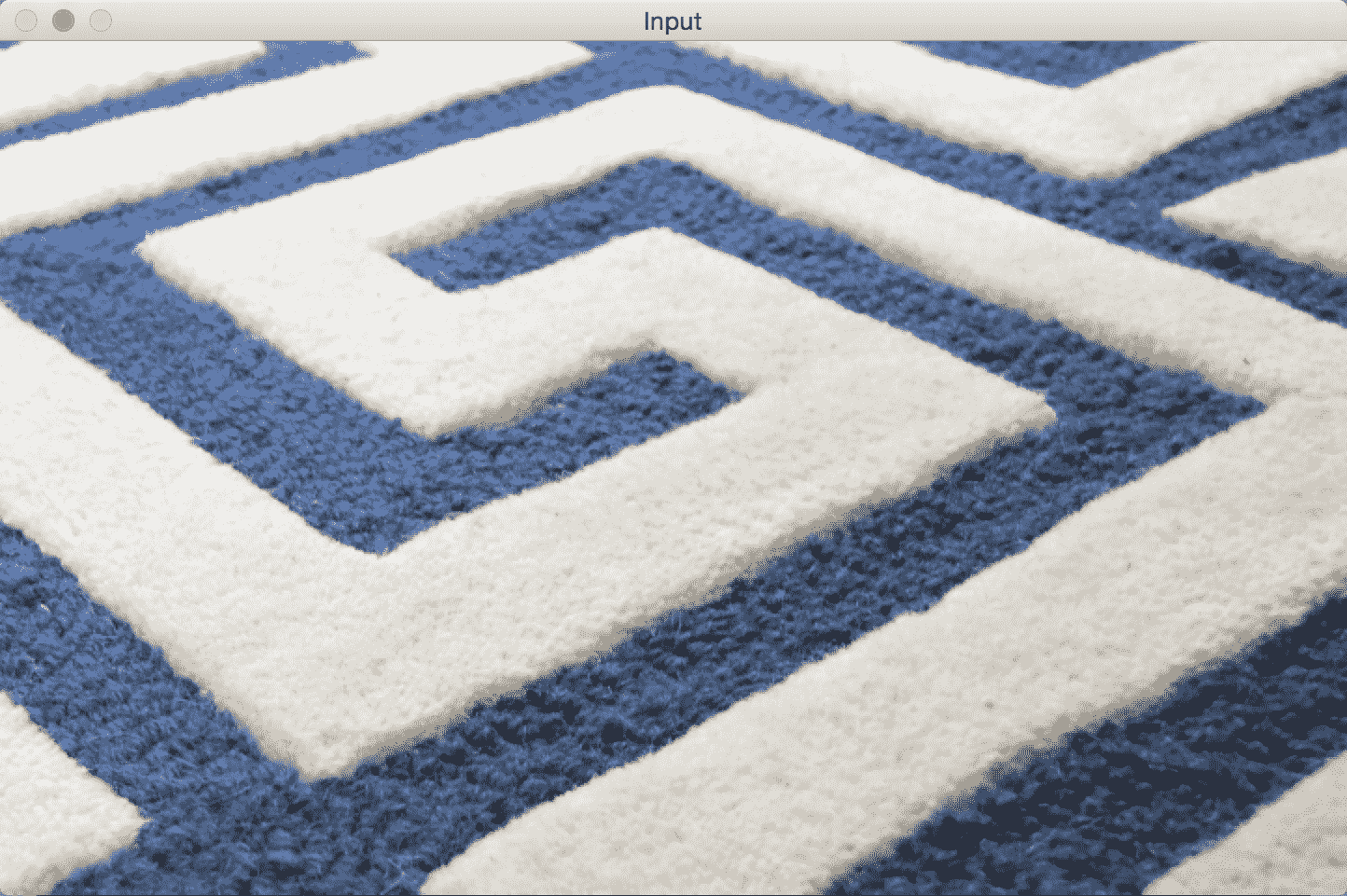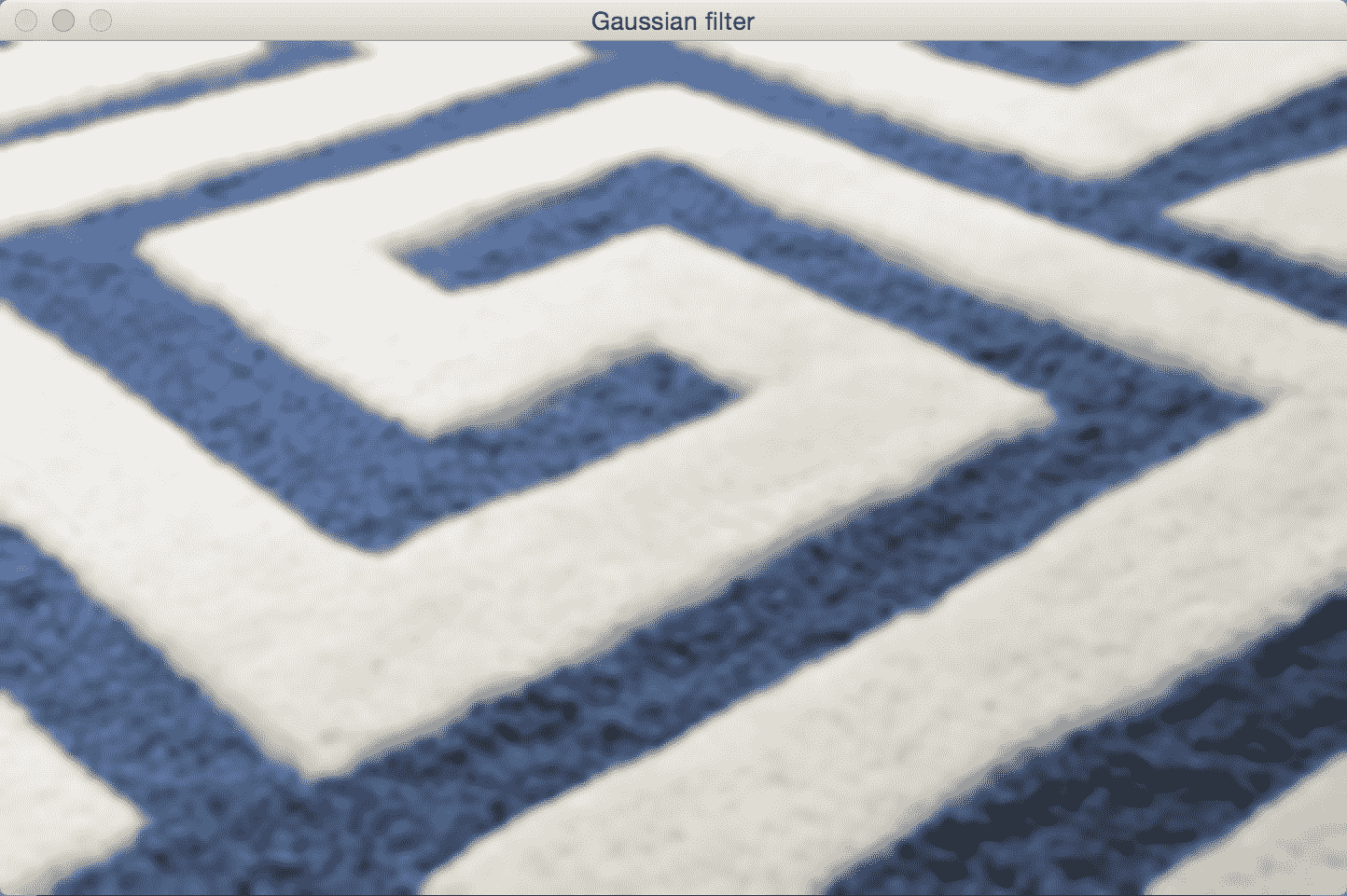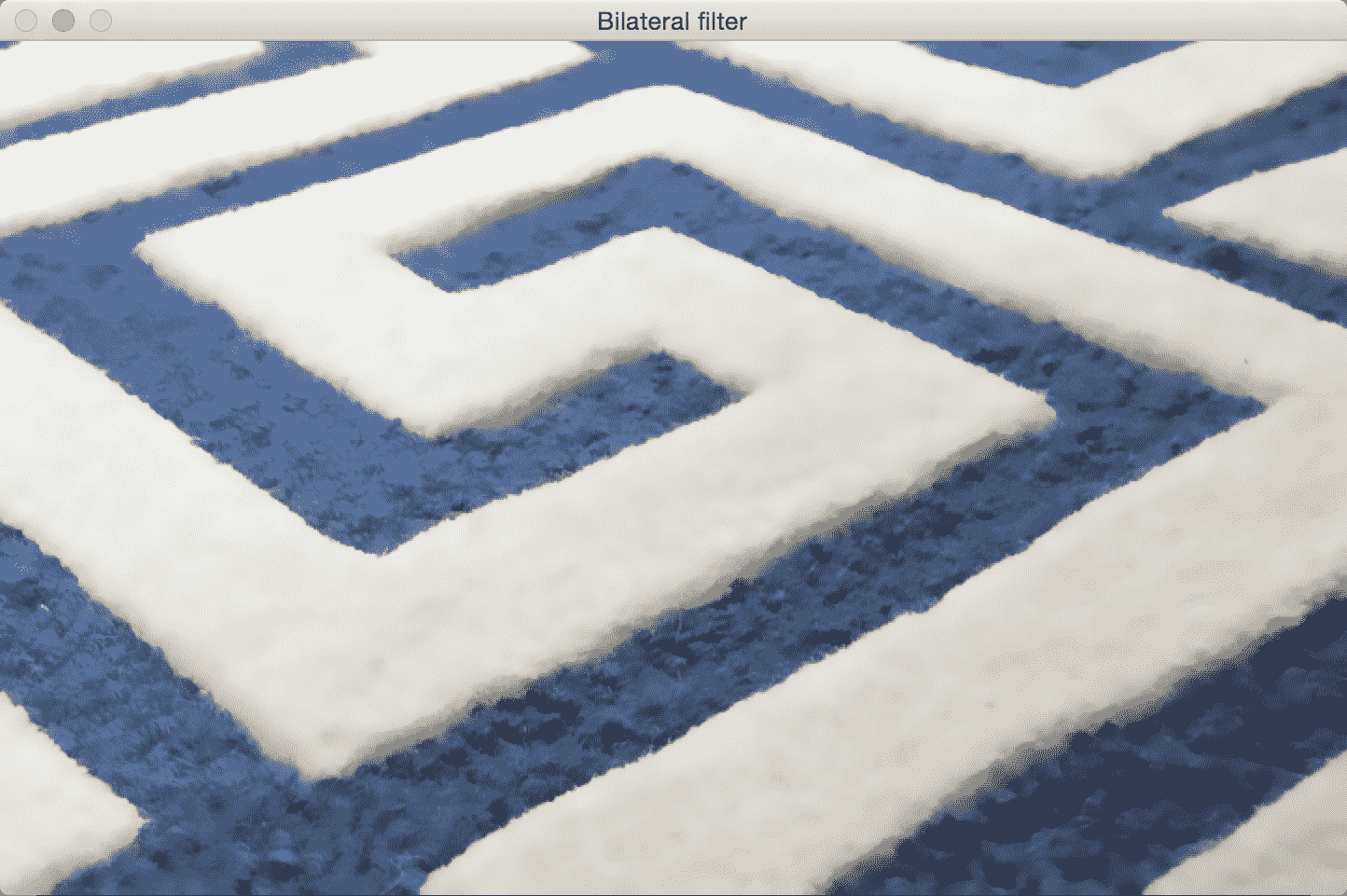import cv2
import numpy as np

img_gaussian = cv2.GaussianBlur(img, (13,13), 0) # Gaussian Kernel Size 13x13
img_bilateral = cv2.bilateralFilter(img, 13, 70, 50)

cv2.imshow('Input', img)
cv2.imshow('Gaussian filter', img_gaussian)
cv2.imshow('Bilateral filter', img_bilateral)
cv2.waitKey()


img_small = cv2.bilateralFilter(img_small, size, sigma_color,
sigma_space)